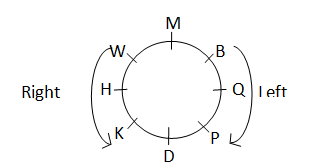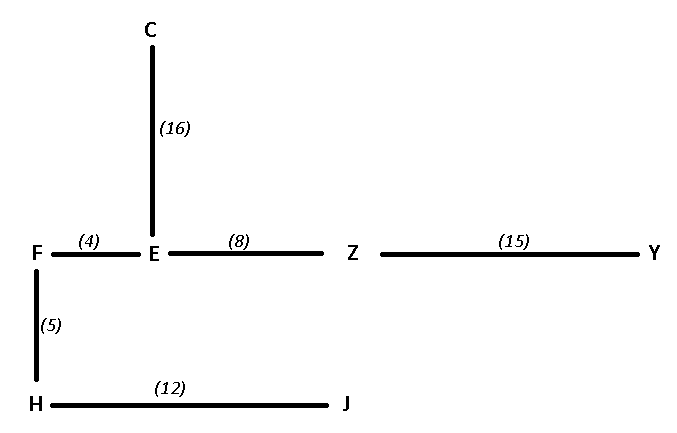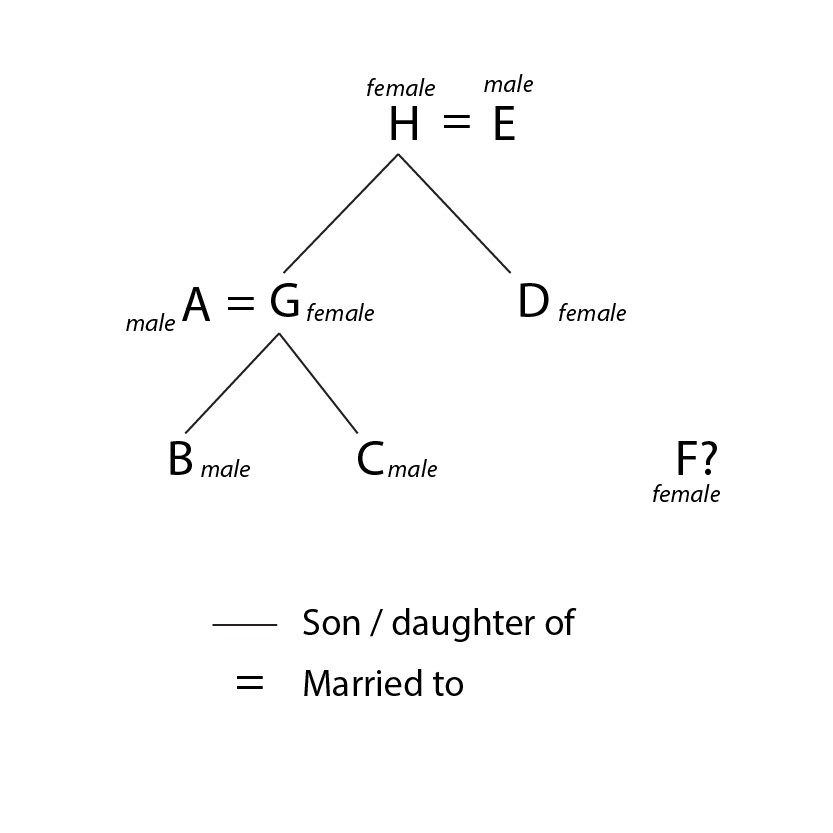0
1054

SBI Clerk Reasoning Expected Questions With Video Explanations PDF

Most expected reasoning questions for SBI clerk 2019 prelims exam download PDF. Go through the video of reasoning questions explanations.

SBI Clerk Topic-Wise Important Questions (Download PDF)

Good number of attempts in SBI Clerk Prelims:

• Reasoning – 30+
• Quant – 25+
• English – 20+
• Total -75+

SBI Clerk Reasoning Expected Questions

Watch the video here (If not loaded below)Instructions

Read the following information carefully and answer the questions which follow.

Eight friends Pawan, Rohit, Sanjay, Tushar, Uday, Vikas, Yash and Zaheer are living in the same building. Each one of them lives on a different floor among floors 1 to 8. Exactly two people live below Yash. Zaheer lives on a floor whose number is odd. Exactly two people live between Zaheer and Rohit, however, Rohit does not live on the top most floor. Pawan lives on a floor whose floor number is either prime or composite. Sanjay and Tushar live on adjacent floors with Tushar living on a higher floor. Sanjay does not live on the floor which is a multiple of both 2 and 3 while Uday does not live on the floor which is a multiple of 3. Only 1 person lives between Yash and Pawan. Zaheer does not live on the second highest floor.

Question 1: Who lives on floor number 7?

a) Sanjay

b) Uday

c) Tushar

d) Yash

e) Cannot be determined

Question 2: Which of the following is definitely true regarding Vikas?

a) Only 3 people are living between Vikas and Rohit

b) Vikas lives immediately above Tushar

c) Vikas lives on floor number 6

d) 2 people live between Vikas and Rohit

e) None of the statements are definitely true

Question 3: What is the floor number on which Tushar lives?

a) 6

b) 5

c) 7

d) 8

e) Cannot be determined

Question 4: How many people live between Pawan and Uday?

a) 1

b) 2

c) 3

d) 4

e) 5

Question 5: Who lives on the floor immediately below Yash?

a) Vikas

b) Rohit

c) Zaheer

d) Pawan

e) Uday

Instructions

Study the following information carefully and answer the questions given below.

B, D, M, K, P, Q, W and H are sitting around a circle facing at the centre. M is to the immediate right of B who is 4th to the right of K. P is 2nd to the left of B and is 4th to the right of W.Q is 2nd to the right of D who is 2nd to the right of H.

Question 6: Who is third to the right of B?

a) W

b) M

c) K

d) H

e) None of these

Question 7: Which of the following represents the immediate neighbours of D?

a) PQ

b) KH

c) PH

d) KQ

e) PK

Question 8: Who is third to the right of W?

a) P

b) D

c) K

d) R

Question 9: Who is second to the left of P?

a) D

b) H

c) K

e) None of these

Question 10: Who is to the immediate left of B?

a) Q

b) P

c) W

e) None of these

Instructions

Study the given information carefully to answer the given questions.
Point E is 16 m to the south of Point C. Point F is 4 m to the west of Point E. Point H is 5 m to the south of Point F. Point J is 12 m to the east of Point H. Point Y is 27 m to the east of Point F. A person walks 15 m from Point Y towards west, reaches Point Z, takes a left turn and reaches Point J.

Question 11: What is the difference of the distance between the points Z, J and points Y, F ?

a) 26 m

b) 22 m

c) 12 m

d) 8 m

e) 20 m

Question 12: If a person walks 20m towards north from Point Z, takes a left turn and walks 8 m, how far will be from Point C ?

a) 2 m

b) 6 m

c) 3 m

d) 4 m

e) 5 m

Question 13: In which direction is Point Z with respect to Point H?

a) Northwest

b) Northeast

c) Southwest

d) East

e) South east

Instructions

In a family of 8 people, G is the mother of B. H has two daughters – G and D. F is H’s only granddaughter. E is D’s father. C is the brother of B. B is A’s son.

Question 14: How many children does D have?

a) 0

b) 1

c) 2

d) Either 0 or 1

e) Either 1 or 2

Question 15: How is D related to C?

a) Uncle

b) Aunt

c) Brother

d) Sister

e) Can’t be determined

Exactly two people live below Yash. Hence, Yash must be living on floor number 3. Now exactly 1 person lives between Yash and Pawan. So Pawan can be at 1 or 5. However, 1 is neither prime nor composite. Hence, Pawan must be at 5. Zaheer lives on an odd numbered floor but not on floor number 7. Hence, Zaheer must be living on floor number 1 (since 3 and 5 are already occupied). Two people live between Zaheer and Rohit. Hence, Rohit must be living at the floor number 4. Sanjay and Tushar live on adjacent floors. So only possibilities are 6,7 or 7, 8. Sanjay does not live on 6. Hence, he must be living at 7 and Tushar must be at 8. Now Uday does not live at 6 so he must be at 2. Thus, Vikas must be at 6. Hence, the final arrangement is as shown below

Exactly two people live below Yash. Hence, Yash must be living on floor number 3. Now exactly 1 person lives between Yash and Pawan. So Pawan can be at 1 or 5. However, 1 is neither prime nor composite. Hence, Pawan must be at 5. Zaheer lives on an odd numbered floor but not on floor number 7. Hence, Zaheer must be living on floor number 1 (since 3 and 5 are already occupied). Two people live between Zaheer and Rohit. Hence, Rohit must be living at the floor number 4. Sanjay and Tushar live on adjacent floors. So only possibilities are 6,7 or 7, 8. Sanjay does not live on 6. Hence, he must be living at 7 and Tushar must be at 8. Now Uday does not live at 6 so he must be at 2. Thus, Vikas must be at 6. Hence, the final arrangement is as shown below

Exactly two people live below Yash. Hence, Yash must be living on floor number 3. Now exactly 1 person lives between Yash and Pawan. So Pawan can be at 1 or 5. However, 1 is neither prime nor composite. Hence, Pawan must be at 5. Zaheer lives on an odd numbered floor but not on floor number 7. Hence, Zaheer must be living on floor number 1 (since 3 and 5 are already occupied). Two people live between Zaheer and Rohit. Hence, Rohit must be living at the floor number 4. Sanjay and Tushar live on adjacent floors. So only possibilities are 6,7 or 7, 8. Sanjay does not live on 6. Hence, he must be living at 7 and Tushar must be at 8. Now Uday does not live at 6 so he must be at 2. Thus, Vikas must be at 6. Hence, the final arrangement is as shown below

Exactly two people live below Yash. Hence, Yash must be living on floor number 3. Now exactly 1 person lives between Yash and Pawan. So Pawan can be at 1 or 5. However, 1 is neither prime nor composite. Hence, Pawan must be at 5. Zaheer lives on an odd numbered floor but not on floor number 7. Hence, Zaheer must be living on floor number 1 (since 3 and 5 are already occupied). Two people live between Zaheer and Rohit. Hence, Rohit must be living at the floor number 4. Sanjay and Tushar live on adjacent floors. So only possibilities are 6,7 or 7, 8. Sanjay does not live on 6. Hence, he must be living at 7 and Tushar must be at 8. Now Uday does not live at 6 so he must be at 2. Thus, Vikas must be at 6. Hence, the final arrangement is as shown below

Exactly two people live below Yash. Hence, Yash must be living on floor number 3. Now exactly 1 person lives between Yash and Pawan. So Pawan can be at 1 or 5. However, 1 is neither prime nor composite. Hence, Pawan must be at 5. Zaheer lives on an odd numbered floor but not on floor number 7. Hence, Zaheer must be living on floor number 1 (since 3 and 5 are already occupied). Two people live between Zaheer and Rohit. Hence, Rohit must be living at the floor number 4. Sanjay and Tushar live on adjacent floors. So only possibilities are 6,7 or 7, 8. Sanjay does not live on 6. Hence, he must be living at 7 and Tushar must be at 8. Now Uday does not live at 6 so he must be at 2. Thus, Vikas must be at 6. Hence, the final arrangement is as shown below

We start by placing M. To find people to one’s right, we move in the anti-clockwise direction and for left in the clockwise direction. With M placed, we can place B. Four places to the left or right will essentially be opposite spot. Hence, we can place K. We can place P with respect to B and W from P. Only three spots remain, so they must be arranged between H-D-Q as shown below.Hence, H is third to the right of B.

We start by placing M. To find people to one’s right, we move in the anti-clockwise direction and for left in the clockwise direction. With M placed, we can place B. Four places to the left or right will essentially be opposite spot. Hence, we can place K. We can place P with respect to B and W from P. Only three spots remain, so they must be arranged between H-D-Q as shown below.Hence, PK are immediate neighbours of D.

We start by placing M. To find people to one’s right, we move in the anti-clockwise direction and for left in the clockwise direction. With M placed, we can place B. Four places to the left or right will essentially be opposite spot. Hence, we can place K. We can place P with respect to B and W from P. Only three spots remain, so they must be arranged between H-D-Q as shown below.D is third to the right of W.

We start by placing M. To find people to one’s right, we move in the anti-clockwise direction and for left in the clockwise direction. With M placed, we can place B. Four places to the left or right will essentially be opposite spot. Hence, we can place K. We can place P with respect to B and W from P. Only three spots remain, so they must be arranged between H-D-Q as shown below.K is second to the left of P

We start by placing M. To find people to one’s right, we move in the anti-clockwise direction and for left in the clockwise direction. With M placed, we can place B. Four places to the left or right will essentially be opposite spot. Hence, we can place K. We can place P with respect to B and W from P. Only three spots remain, so they must be arranged between H-D-Q as shown below.Hence, Q is to the immediate left of B.

Y is 27m to the east of point F. When one walks 15m to the west from point Y, he reaches Z.

=> Z is 12m to the east of point F and 8m to the east of point E.

Distance between F and H is 5m which is also equal to the distance between Z and J.

Based on the above conditions, these points when plotted on a graph will be :

The numbers in the brackets is the distance between any two points.Distance between Z & J = 5

Distance between F & Y = 4+8+15 = 27

Required difference = 27-5 = 22 m

Thus, Ans – (B)

Y is 27m to the east of point F. When one walks 15m to the west from point Y, he reaches Z.

=> Z is 12m to the east of point F and 8m to the east of point E.

Distance between F and H is 5m which is also equal to the distance between Z and J.

Based on the above conditions, these points when plotted on a graph will be :

The numbers in the brackets is the distance between any two points.If a person walks 20 m north from point Z and then take left turn and walk 8 m .

He will be = 20-16 = 4m to the north of point C.

Thus, Ans – (D)

Y is 27m to the east of point F. When one walks 15m to the west from point Y, he reaches Z.

=> Z is 12m to the east of point F and 8m to the east of point E.

Distance between F and H is 5m which is also equal to the distance between Z and J.

Based on the above conditions, these points when plotted on a graph will be :

The numbers in the brackets is the distance between any two points.Clearly, Z is to the north-east of H.

Thus, Ans – (B)

From the information given, we can draw a family tree as shown below.We don’t know if F is G’s or D’s daughter. Hence, D has either 0 or 1 child.